This node creates a half-sphere or semi-ellipsoid plot.

To add a Half-sphere node, right-click in the Graph Editor and select Create Node  > Terrain Generation  > Half-sphere.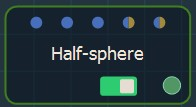Double click on the node to open its parameters: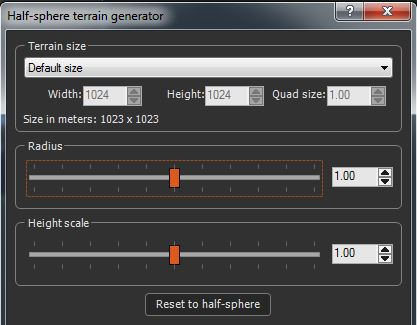### Setting the terrain size

• To change the terrain size, edit the Width and Height fields in number of vertices or select a size preset from the list.
• To change the quad size, edit the Quad size field in meters.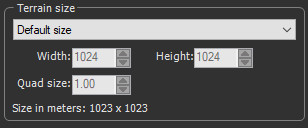Use the Radius slider to set the surface covered by the half-sphere: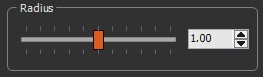• For a value of 1, the diameter of the half-sphere is exactly the size of the terrain, taking into account the smallest dimension of the terrain if it is not square.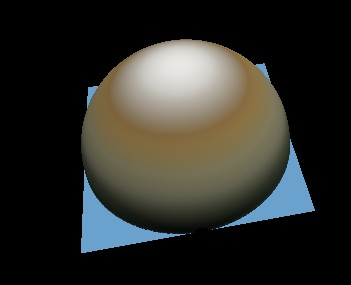• For a value less than 1, the half-sphere is contained entirely inside the terrain.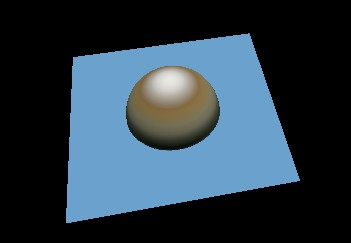• For a value greater than 1, the half-sphere is larger than the terrain.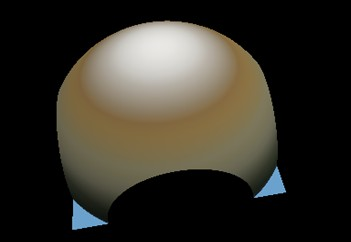Click OK to validate.

### Setting the half-sphere height scale

Use the Height scale slider to set the maximum height of the terrain: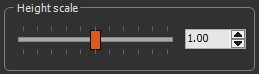• A value of 1 is the default value of the half-sphere.
• For a value less than 1, the half-sphere is flattened.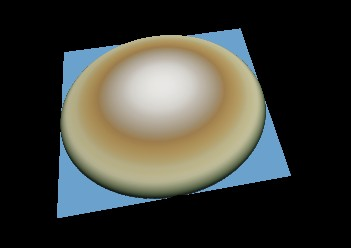• For a value greater than 1, the half-sphere is stretched.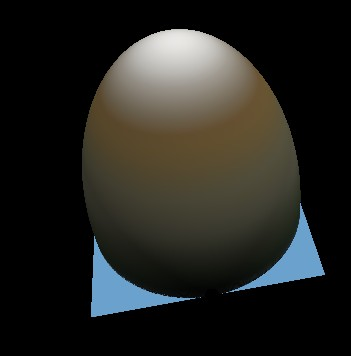Click OK to validate.

### Working with connectors

The Half-sphere node has two connectors to drive a parameter using a formula or a mask.

• The first connector allows you to drive the radius of the half-sphere.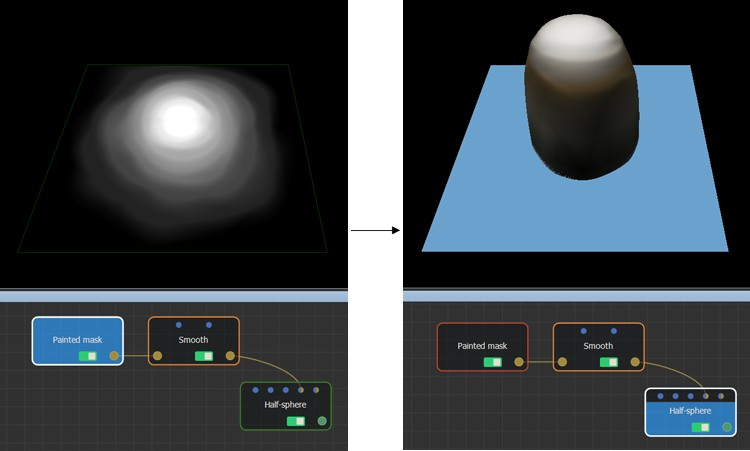• The second connector allows you to drive the height scale of the half-sphere.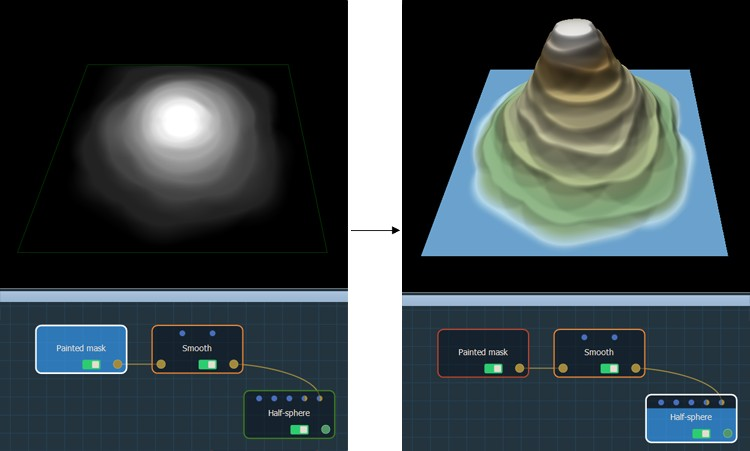### Resetting the default values

The Reset to half-sphere button resets the half-sphere to the default values.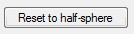### Sample project

In the sample project below, two Slope terrain generators, one with a maximum height of 0 and the other with the inclination angle set to 200, are composed with a Full composition node.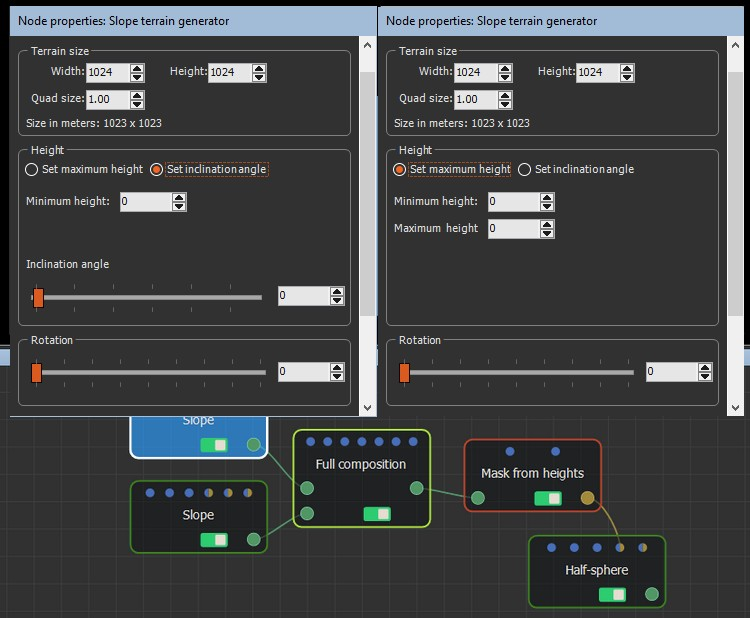The Full composition output is linked to a Mask from heights node, which has a gradient slope with a minimum height of 0 and a maximum height of 100.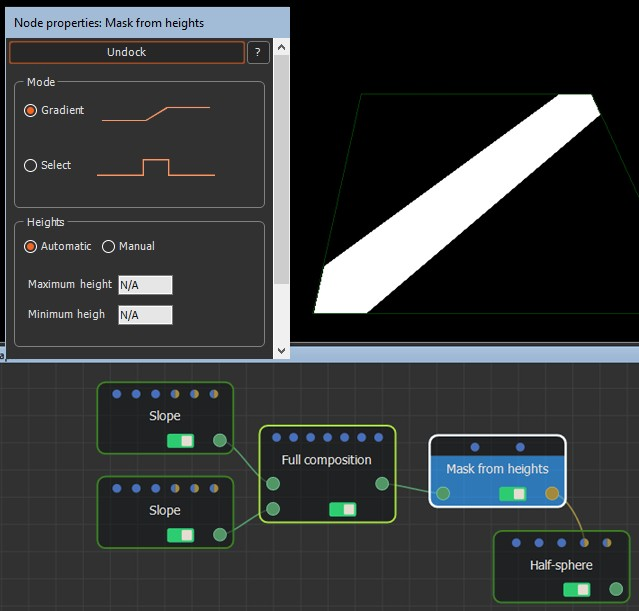The mask's output is linked to the Half-sphere's optional connector. The result is shown by clicking on the Half sphere node.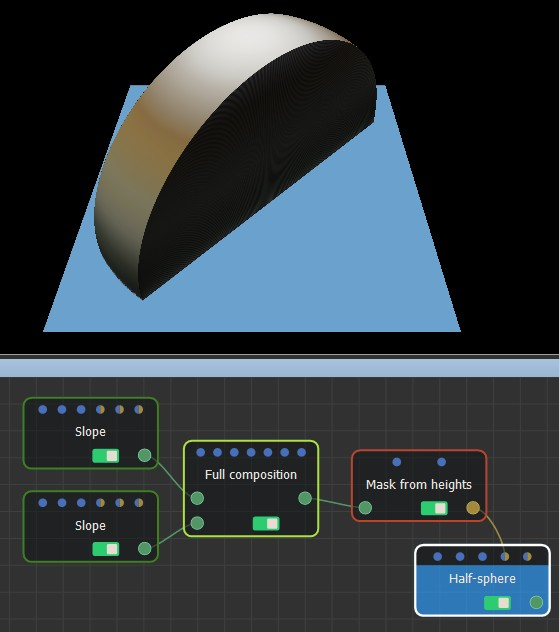### Parameters

Parameter Use
Terrain size See Part common to all generator nodes
Radius Determines the surface covered by the half-sphere
Height scale Determines the maximum height of the terrain